﻿ Reliability Level of Methane Gas Production Dependence on Organic Loading Rate and Hydraulic Retention Time during Biodegradation of Fruit Wastes

### Reliability Level of Methane Gas Production Dependence on Organic Loading Rate and Hydraulic Retenti...

C. Nwoye, A. O. Agbo, K. C. Nnakwo, E. M. Ameh, C. C. NwogbuOPEN ACCESSPEER-REVIEWED

## Reliability Level of Methane Gas Production Dependence on Organic Loading Rate and Hydraulic Retention Time during Biodegradation of Fruit Wastes

C. Nwoye1,, A. O. Agbo2, K. C. Nnakwo1, E. M. Ameh2, C. C. Nwogbu3

1Department of Metallurgical and Materials Engineering, Nnamdi Azikiwe University, Awka, Nigeria

2Department of Metallurgical and Materials Engineering, Enugu State University of Science & Technology, Enugu, Nigeria

3Science Technical Vocation Schools Management Board

### Abstract

Assessment evaluation of the reliability level of methane gas production dependence on organic loading rate and hydraulic retention time during biodegradation of fruit wastes was carried out. A two-factorial linear model was derived and validated for the predictive analysis and evaluation. The validity of the model; ζ = - 0.3127 (ln ϑ - ln ɤ) - 0.2014 was rooted on the core model expression ζ + 0.2014 = - 0.3127(ln ϑ - ln ɤ) where both sides of the expression are correspondingly approximately equal. Regression model was used to generate results of methane gas yield, and its trend of distribution was compared with that from derived model as a means of verifying its validity relative to experimental results. The results of this verification show very close dimensions of aligned areas designating methane gas yield, which precisely translated into significantly similar trend of data point’s distribution for experimental (ExD), derived model (MoD) and regression model-predicted (ReG) results. Evaluations from generated results indicated that methane gas yield per unit hydraulic retention time and organic load mass as obtained from experiment, derived model & regression model were 0.0242, 0.0239 & 0.0209 m3 kg -1 VS d-1 and 0.0064, 0.0063 & 0.0055 (m3 kg -1)2 respectively. Standard errors incurred in predicting the methane gas yield for each value of the hydraulic retention time and organic loading rate considered as obtained from experiment, derived model & regression model were 0.1032, 0.1042, & 0.0011 % and 0.1237, 0.1220 & 2.5x10-5 % respectively. Deviational analysis indicates that the maximum deviation of model-predicted methane gas yield from the experimental results is less than 22%. This translates into over 78% operational confidence and reliability level for the derived model as well as over 0.78 reliability coefficient for the methane gas production dependence on organic loading rate and hydraulic retention time.

### At a glance: Figures

1234
Prev Next

• Nwoye, C., et al. "Reliability Level of Methane Gas Production Dependence on Organic Loading Rate and Hydraulic Retention Time during Biodegradation of Fruit Wastes." International Journal of Environmental Bioremediation & Biodegradation 1.2 (2013): 66-72.
• Nwoye, C. , Agbo, A. O. , Nnakwo, K. C. , Ameh, E. M. , & Nwogbu, C. C. (2013). Reliability Level of Methane Gas Production Dependence on Organic Loading Rate and Hydraulic Retention Time during Biodegradation of Fruit Wastes. International Journal of Environmental Bioremediation & Biodegradation, 1(2), 66-72.
• Nwoye, C., A. O. Agbo, K. C. Nnakwo, E. M. Ameh, and C. C. Nwogbu. "Reliability Level of Methane Gas Production Dependence on Organic Loading Rate and Hydraulic Retention Time during Biodegradation of Fruit Wastes." International Journal of Environmental Bioremediation & Biodegradation 1, no. 2 (2013): 66-72.

 Import into BibTeX Import into EndNote Import into RefMan Import into RefWorks

### 1. Introduction

The growing need for a sustainable application of renewable energy for industrial growth and sustainability of environmental remediation from waste pollution has resulted to series of successful conversion of animal dung, fruits and vegetable wastes to biogases used for heat and power generation.

Optimum biogas production  has been achieved at C : N ratio, 25-30 : 1. Research has reported that the optimum loading rate of the feedstock varied as per its nature, and likewise HRT varied as per the loading rate. Earlier report indicate that an HRT of 14 days was optimum for biogas production from cow dung. It was found  that the optimum retention time for the production of biogas from cattle dung is 15 d HRT. They stated that this retention time was the best for maximum production of biogas from cow dung. They further observed that shorter HRT resulted in accumulation of VFA, whereas at HRT longer than 15 d the digester components were not fully utilized. Studies  on the influence of different TS content of biogas production revealed that optimum production was observed at 8 per cent TS. However, the methane content of the gas produced was not found to vary significantly with varying levels of TS. Hence, they suggested that high TS content of 14% cattle dung (2 : 1 dilution) can be followed in areas during the time of water scarcity rather than discontinuing the feeding of the digester.

Various substrates have been explored for biogas production. During biogas production, it has been found  that the two most important parameters in the selection of particular plant feed stocks are the economic considerations and the yield of methane for fermentation of that specific feedstock. They reported that the highest yield of methane was obtained from root crops followed by forage grasses, and fresh-water aquatics, following their assessment of the methane yield from fresh-water aquatics, forage grasses, roots and tubers, and marine species. Marine species were found  to give the lowest yield of methane. Methane yield and kinetics have been found to be generally higher in leaves than in stems . This concept was confirmed [9, 10], using Ipomoea fistulosa as substrate.

Observations on biogas production from food industry wastes  and from different biomass  have shown that pretreatment of feedstock improved the biogas yield and methane content from biomass-fed digesters. It was observed  that though the agricultural residues, such as straw, need to be reduced to a particle size of 0.4 mm for better biogas production, succulent leaves such as Mirabilis sp., Ipomoea fistulosa, etc. can be fed without any shredding. Evaluation of the biogas potential of Gliricidia sp., Albizia sp., and Parthenium sp. in plug flow digester has been carried out . Results from this evaluation indicate that biogas yield from these feedstocks improved with specific inoculum. It was reported  that pretreatment with NaOH increased the gas production from parthenium-fed digesters (0.46 m3 m 3 d 1 and the methane yield was 0.11 m3 kg 1 VS). In previous reports [15, 16] water hyacinth was used as alternate substrate together with cattle waste for biogas digesters. Researchers  have developed horizontal plug flow (PF) digester and solid state stratified bed (SSB) digester for bio-methanation of biomass and observed that SSB performed better in terms of stability and gas production.

In the case of fruit wastes, It has been reported  that tomato, mango, pineapple, lemon, and orange processing waste, yielded 0.62, 0.56, 0.77, 0.72 and 0.63 m3 of biogas/kg of VS respectively. It was established  that Mango peel supplemented with urea to adjust the C : N ratio to 20–30 : 1 resulted in the stability of the digester. Further studies  reported that addition of nitrogen in the form of silkworm waste and oilseed extracts, such as neem and castor, increased the methane content. Investigation  on the influence of successive addition of fruit and vegetable solid wastes on the performance of biogas digester shows that the digester was stable at a loading rate of 3.8 kg VS m–3 d 1. They further observed that no noticeable changes in the rates and yields of biogas occurred as a result of minor manipulation in nutritional and operational parameters which practically helped in the functioning of the digester fed with different fruits and vegetable wastes (mango, pineapple, tomato, jack fruit, banana, and orange) for a considerably long time.

Successful studies  carried out on Pilot plant (of volumetric capacity 1.5 m3 and digester type KVIC) with mango peel significantly showed that supplementation with essential nutrients improved the digestibility of feedstock, yielding as high as 0.6 m3/kg VS with a biogas content of 52% at a loading rate of 8–10%. Also, addition of sugarcane filter mud at a rate of 200 g/4 kg of mango peel in 1.5 m3 digester increased biogas yield substantially with a methane content of 60%. It was also reported  that addition of extract of nirmali seeds, hybrid beans, black gram, and guar gum seeds (as additives) at 2–3% level increased the biogas production significantly. This increment was attributed to the galactomannan constituent of the leguminous seeds which increased the floc formation, thereby retaining the organisms in the digester.

A model was developed  for predictive analysis of methane gas yield during bio-treatment of fruit wastes in the digester. The research was aimed at producing biogas for heat and electricity generation. It was also found that the validity of the model expressed as: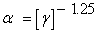(1)

was rooted on the core expression (Log α)/N = Log (γ)-1 where both sides of the expression are correspondingly approximately equal. The overall validity of the derived model was established using the 4th Degree Model Validity Test Technique (4th DMVTT) which are computational, graphical, statistical and deviational analysis. Computational analysis of the model-predicted and experimental results indicates that methane gas yield per unit organic loading rate are - 0.0421 and- 0.0395 (VS)2 d-1 as obtained from experiment and derived model respectively. The graphical analysis, apart from showing very close alignment of curves from both experimental and model-predicted results, indicates 0.9923 and 1.0000 as the correlation between methane yield and organic loading rate as obtained from experiment and derived model are respectively. Statistical analysis of the results indicate that the variance and standard deviation as obtained from experiment and derived model are 9.1004 x 10-4 and 0.0302 as well as 8.0934 x10-4 and 0.0284 respectively, indicating proximate agreement. Deviational analysis indicates that the maximum deviation of the model-predicted methane yield from the corresponding experimental value is less than 6%.

An assessment evaluation of methane gas yield was carried out  based on the hydraulic retention time (HRT) of the feed stock (waste fruits) undergoing biotreatment in the digester. The assessment was carried out using a derived and validated model expressed as;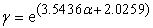(2)

The model depicts an exponential relationship between methane yield and the HRT. Statistical analysis of the model-predicted and experimental gas methane yield for each value of HRT considered shows a standard error of 0.0081 and 0.0114% respectively. Furthermore, the precise correlation between methane yield and HRT as obtained from derived model and experimental results were evaluated as 0.9716 and 0.9709 respectively. Methane gas yield per unit HRT as obtained from derived model and experiment are 0.0196 and 0.0235 (m3 kg−1 VS) days−1 respectively.

Deviational analysis indicates that the maximum deviation of the model-predicted methane yield from the corresponding experimental value is less than 16%. It was also found that the validity of the model is rooted on the expression 0.2822 lnγ = α + 0.5717 where both sides of the expression are correspondingly approximately equal.

The present work aims at assessing the reliability level of methane gas production dependence on organic loading rate and hydraulic retention time during biodegradation of fruit wastes. An empirical model will be derived, validated and used for the predictive assessment. The overall fundamental need of intensively studying about development of models for evaluation of the reliability level of methane gas production is just to avoid carrying out experimental works all the time in order to ascertain the best process parameters values that gives optimum yield. On considering a derived model as well as the initial and boundary conditions, a new output value could be predicted without carrying out further experiment by just substituting any process parameter value within the range of these conditions into the model.

### 2. Materials and Methods

A weighed quantity of prepared fruit wastes was put in the digested containing the appropriate microbes. Details of the experimental procedure and associated process conditions are as stated in the past report .

#### Table 1. Variation of methane gas production with organic load mass, loading rate and hydraulic retention time 

Where

(₰) = Organic load mass (kg VS m-3 )

(ɤ) = Hydraulic retention time (days)

(ζ) = Methane gas production (m3 kg-1 VS)

2.1. Model Formulation

Experimental data obtained from research work  were used for this work. Computational analysis of the data  shown in Table 1, gave rise to Table 2 which indicate that;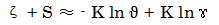(3)

Introducing the values of S and K into equation (3) reduces it to;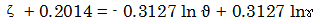(4)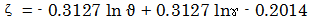(5)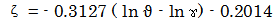(6)

S = 0.2014 and K = 0.3127 are empirical constants (determined using C-NIKBRAN 

### 3. Boundary and Initial Condition

Consider prepared fruit wastes (in a digester) interacting with microbes. The digester atmosphere was not contaminated i.e (free of unwanted gases and dusts). Range of organic loading rate and hydraulic retention time used: 3.8-7.6 kgVS m-3 d-1 and 8-20days respectively. Mass of wastes used, resident time, treatment temperature, growth rate of microbes and other process conditions are as stated in the experimental technique .

The prevailed boundary conditions are: anaerobic atmosphere to enhance bacterial action on the wastes (since the digester was air-tight closed).

At the bottom of the particles, a zero gradient for the gas scalar are assumed and also for the gas phase at the top of the waste particles. The biodegraded waste is stationary. The sides of the waste particles are taken to be symmetries.

### 4. Results and Discussion

It is pertinent to state that the proposed model is very suitable for explaining the bio-degradation of the fruit waste, due to bacteria. This is because the model was formulated using results generated from a previously carried out bio-degradation process , as its initial and boundary conditions. And so, what the derived model does is just to empirically interpret the process.

4.1. Model Validation

Equation (3) is the derived model.

#### Table 2. Variation of ζ + 0.2014 with - 0.3127 ln ϑ + 0.3127 lnɤ

The validity of the model is strongly rooted on equation (4) where both sides of the equation are correspondingly approximately equal. Table 2 also agrees with equation (4) following the values of ζ + 0.2014 and - 0.3127 ln ϑ + 0.3127 lnɤ evaluated from the experimental results in Table 1. Furthermore, the derived model was validated by comparing the methane gas yield predicted by the model and that obtained from the experiment . This was done using the 4th Degree Model Validity Test Techniques (4th DMVTT); computational, graphical, statistical and deviational analysis .

Figure 1. Coefficient of determination between methane gas yield and organic loading rate as obtained from experiment 
Figure 3. Coefficient of determination between methane gas yield and hydraulic retention time as obtained from experiment 
Figure 4. Coefficient of determination between methane gas yield and hydraulic retention time as predicted by derived model
Figure 5. Comparison of methane gas yields (relative to organic loading rate) as obtained from experiment  and derived model (Trend line)
Figure 6. Comparison of methane gas yields (relative to hydraulic retention time) as obtained from experiment  and derived model (Trend line)
Figure 7. Comparison of methane gas yields (relative to organic loading rate) as obtained from experiment  and derived model (line distribution)
Figure 8. Comparison of methane gas yields (relative to hydraulic retention time) as obtained from experiment  and derived model (line distribution)
Figure 9. Comparison of areas covered by methane gas yield (relative to organic loading rate) as obtained from experiment , derived model and regression model
Figure 10. Comparison of areas covered by methane gas yield (relative to hydraulic retention time) as obtained from experiment , derived model and regression model
4.2. Statistical Analysis

Standard Error (STEYX)

The standard errors incurred in predicting methane gas yield for each value of the hydraulic retention time and organic loading rate considered as obtained from experiment and derived model were 0.1032 & 0.1042% and 0.1237 & 0.1220 % respectively. The standard error was evaluated using Microsoft Excel version 2003.

Correlation (CORREL)

The correlation coefficient between methane gas yield and hydraulic retention time & organic loading rate were evaluated from the results of derived model and experiment, considering the coefficient of determination R2 from Figure 1, Figure 2, Figure 3, Figure 4, was calculated using Microsoft Excel version 2003.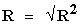(7)

The evaluated correlations are shown in Table 3. These evaluated results indicate that the derived model predictions are significantly reliable and hence valid considering its proximate agreement with results from actual experiment.

#### Table 4. Comparison of the correlations evaluated from derived model predicted and ExD results based on organic loading rate

4.3. Graphical Analysis

Comparative graphical analysis of Figure 5, Figure 6, Figure 7, and Figure 8 show very close alignment of the curves from the experimental (ExD) and model-predicted (MoD) methane gas yields. Furthermore, the degree of alignment of these curves is indicative of the proximate agreement between both experimental and model-predicted methane gas yields.

4.4. Comparison of Derived Model with Standard Model

The validity of the derived model was also verified through application of the regression model (Reg) (Least Square Method using Excel version 2003) in predicting the trend of the experimental results. Comparative analysis of Figure 9 and Figure 10 shows very close dimensions of aligned areas covered by methane gas yield, which precisely translated into significantly similar trend of data point’s distribution for experimental (ExD), derived model (MoD) and regression model-predicted (ReG) results of methane gas yield.

Also, the calculated correlations (from Figure 9 and Figure 10) between methane gas yield and organic loading rate & hydraulic retention time for results obtained from regression model gave 0.9999 & 1.0000 respectively. These values are in proximate agreement with both experimental and derived model-predicted results. The standard errors incurred in predicting methane gas yield for each value of the hydraulic retention time and organic loading rate considered as obtained from regression model were 0.0011 and 2.5 x 10-5% respectively.

Computational Analysis

Comparative computational analysis of the experimental and model-predicted methane gas yield was carried out to ascertain the degree of validity of the derived model. This was done by comparing results of evaluated methane gas yield per unit hydraulic retention time and organic loading rate as obtained from experimental and derived model within organic loading rate and hydraulic retention time range: 3.8-7.6 kgVS m-3 d-1 and 8-20days respectively.

Methane gas yield per unit organic load mass ζ / ₰ (m3 kg -1)2 was calculated from the equation;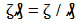(8)

Re-written as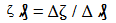(9)

Equation (9) is detailed as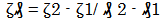(10)

Where

Δζ = Change in the methane gas yield ζ 2, ζ 1 at two organic load masses ₰ 2 - ₰ 1.

Considering the points (76, 0.32) & (30.4, 0.03), (76, 0.3179) & (30.4, 0.0314) and (76,0.2525) & (30.4, 0.0016) as shown in Table 1 and Figure 1, Figure 2, Figure 5, Figure 7 & Figure 9 and then designating them as (ζ1, ₰1) & (ζ22) for experimental, derived model and regression model predicted results respectively, and then substituting them into equation (10), gives the slopes: 0.0064, 0.0063, 0.0055 (m3 kg-1)2 respectively as their corresponding methane gas yield per unit organic load mass.

Methane gas yield per unit hydraulic retention time ζ / ɤ (m3 kg-1VSd-1) was similarly calculated by substituting into equation (10) points (20, 0.32) & (8, 0.03), (20, 0.3179) & (8, 0.0314) and (20,0.2525) & (8, 0.0016) from Table 1 and Figure 3, Figure 4, Figure 6, Figure 8 & Figure 10 as (ζ1, ɤ1) & (ζ22) for experimental, derived model and regression model predicted results respectively. This substitution gave slopes: 0.0064, 0.0063 and 0.0055 m3 kg -1 VS d-1 respectively as their corresponding methane gas yield per unit hydraulic retention time. The proximity between values in each result set indicates significantly high validity level for the derived model.

Deviational Analysis

Analysis of the methane gas yields precisely obtained from experiment  and derived model shows low deviations on the part of the model-predicted values relative to values obtained from the experiment. This is attributed to the fact that the surface properties of fruit wastes and the physiochemical interactions between the fruit wastes and the infesting microbes which played vital roles during the biogas production process  were not considered during the model formulation. This necessitated the introduction of correction factor, to bring the model-predicted methane gas yield to those of the corresponding experimental values.

The deviation Dv, of model-predicted methane gas yield from the corresponding experimental result was given by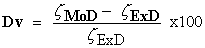(11)

Where

ζExD and ζMoD are methane gas yield evaluated from experiment and derived model respectively.

The correction factor took care of the negligence of operational contributions of surface properties of the fruit wastes and the physiochemical interactions between the fruit wastes and infesting microbes which actually played vital role during the methane gas production process. The model predicted results deviated from those of the experiment because these contributions were not considered during the model formulation. Introduction of the corresponding values of Cf from equation (9) into the model gives exactly the corresponding experimental methane gas yield.

#### Table 5. Deviation of model-predicted Methane gas yield (from that of experiment)

Deviational analysis of Table 5 indicates that the precise maximum deviation of model-predicted methane gas yield from the experimental results is less than 22%. This translates into over 78% operational confidence and reliability level for the derived models and well over 0.78 reliability coefficient for the methane gas production dependence on organic loading rate and hydraulic retention time.

Consideration of equation (8) and critical analysis of Table 5 shows that the least and highest magnitudes of deviation of the model-predicted methane gas yield (from the corresponding experimental values) are - 0.66 and - 21.5%. Table 5 and Figure 1 to Figure 8 indicates that these deviations correspond to methane gas yields: 0.3179 and 0.0314 m3 kg -1 VS; organic loading rates: 3.8 and 7.6 kg VS m-3 d-1 as well as hydraulic retention times: 20 and 16 days respectively.

Correction factor, Cf to the model-predicted results was given by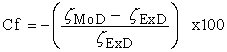(12)

Critical analysis of Table 5 indicates that the evaluated correction factors are negative of the deviation as shown in equations (11) and (12). Table 5 also shows that the least and highest correction factor (to the model-predicted methane gas yield) are 0.66 and 21.5%. Table 5 and Figure 1 to Figure 8 indicate that these highlighted correction factors correspond to methane gas yields: 0.3179 and 0.0314 m3 kg -1 VS; organic loading rates: 3.8 and 7.6 kg VS m-3 d-1 as well as hydraulic retention times: 20 and 16 days respectively.

It is important to state that the deviation of model predicted results from that of the experiment is just the magnitude of the value. The associated sign preceding the value signifies that the deviation is a deficit (negative sign) or surplus (positive sign).

### 5. Conclusion

  Mital, K. M. (1996). Biogas Systems: Principles and Applications, New Age International (P) Limited Publishers, New Delhi, p. 412.In article  Ramasamy, K. (1998). in Renewable Energy – Basics and Technology (ed. Gupta, C. L.), Auroville Foundation and Solar Agni International, Pondicherry, pp. 239-271.In article  Yeole, T. Y. and Ranade, D. R. (1992). Alternative Feedstocks for Biogas, 10-16.In article  Gadre, R. V., Ranade, D. R. and Godbole, S. H. (1990) Indian J. Environ. Hlth, 32, 45-49.In article  Ranade, D. R., Nagarwala, N. N., Dudhbhate, J. A., Gadre, R. V. and Godbole, S. H. (1990). Indian J. Environ. Hlth, 32, 63-65.In article  Smith, W. H., Wilkie, A. C. and Smith, P. H. (1992). TIDE, 2, 1-20.In article  Smith, P. H., Bordeaux, F. M., Wilkie, A., Yang, J., Boone, D., Mah, R. A., Chynoweth, D. and Jerger, D. (1998). In Methane from Biomass: A Systems Approach (eds Smith, W. H. and Frank, J. R.), Elsevier Applied Science, London, p. 500.In article PubMed  Chynoweth, D. P., Turik, C. E., Owens, J. M., Jerger, D. E. and Peck, M. W. (1993). Biomass Bioenergy, 5, 9-111.In article CrossRef  Sharma, S. K., Mishra, I. M., Sharma, M. P. and Saini, J. S. (1998). Biomass, 17, 251-263.In article CrossRef  Sharma, S. K., Saini, J. S., Mishra, I. M. and Sharma, M. P. (1989). Biol. Wastes, 28, 25-32.In article CrossRef  Krishnanand, K. (1994). Indian Food Ind., 13, 33-35.In article  Gunaseelan, V. N. (1997). Biomass Bioenergy, 13, 83-114.In article CrossRef  Ramasamy, K., Ilamurugu, K., Sahul Hameed, M. and Maheswari, M. (1995). Tech. Bull., 5, 36.In article  Gunaseelan, V. N. (1994). Biomass Bioenergy, 6, 391-398.In article CrossRef  Deshpande, P., Sarnaik, S., Godbole, S. H. and Wagle, P. M. (1979). Curr. Sci., 48, 490-492.In article  -Mallick, M. K., Singh, U. K. and Ahmad, N. (1990). Biol. Wastes, 31, 315-319.In article CrossRef  -Chanakya, H. N., Ganguli, N. K., Anand, V. and Jagadeesh, K. S. (1995). Energy Sustain. Dev., 1, 43-46.In article  Anonymous, (1989). Final report submitted to Department of Non-Conventional Energy Sources, Government of India, New Delhi.In article  Viswanath, P., Devi, S. and Krishnanand, Biores. Technol., 1992, 40, 43-48.In article CrossRef  Nwoye, C. I., and Nwabanne, J. T. (2013). Empirical analysis of methane gas yield dependence on organic loading rate during microbial treatment of fruit wastes in digester. Advances in Applied Science Research, 2013, 4(1):308-318.In article  Nwoye, C. I., Asuke, F., Ijomah, A., Obiorah, S. (2012). Model for Assessment Evaluation of Methane Gas Yield Based on Hydraulic Retention Time during Fruit Wastes Biodigestion. Journal of Minerals and Materials Characterization and Engineering, 11: 947-952.In article  Nwoye, C. I., (2008). Data Analytical Memory; C-NIKBRAN.In article PubMed